# Geometric Series

A geometric series is the sum of terms of a geometric sequence.

# Example

A geometric sequence begins:The sum of the first seven terms is:.

Again, because there are only seven terms, it isn’t unreasonable to go ahead and add them together. But let’s do it in a way that can be generalised into a formula.

First, lets give the sum a name. As we are adding seven terms, we call the sum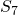.Next, we multiply both sides by the common ratio which is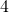.Then we subtractfrom: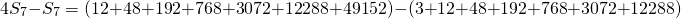Notice that on the left hand side, we just have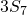, and on the right hand side all but two of the terms cancel out.Simplifying to:This is a tidy calculation to preform: we find that.

# Generalise

To generalize, let’s write the first seven terms of a geometric sequence:Notice that the first term is not multiplied by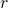and that the last term is theterm.

Now, if we multiply both sides by the common ratiowe have: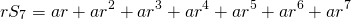Subtracting like we did in the example above we haveAgain, all but two terms on the right hand side cancel out.Let’s divide through by:Now take out the common factoron the numerator:Finally, we are using 7 terms but we could be adding any number of terms, sayterms. Let’s replace the 7 with:And there is the formula.

Notice that to use this formula, it is not necessary to write out the terms of the sequence. The information required is, the number of terms;the first term andthe common ratio.

# Practice

Find eleven questions to practice this math at the end of this mathisfun page.

Next: Sigma Notation